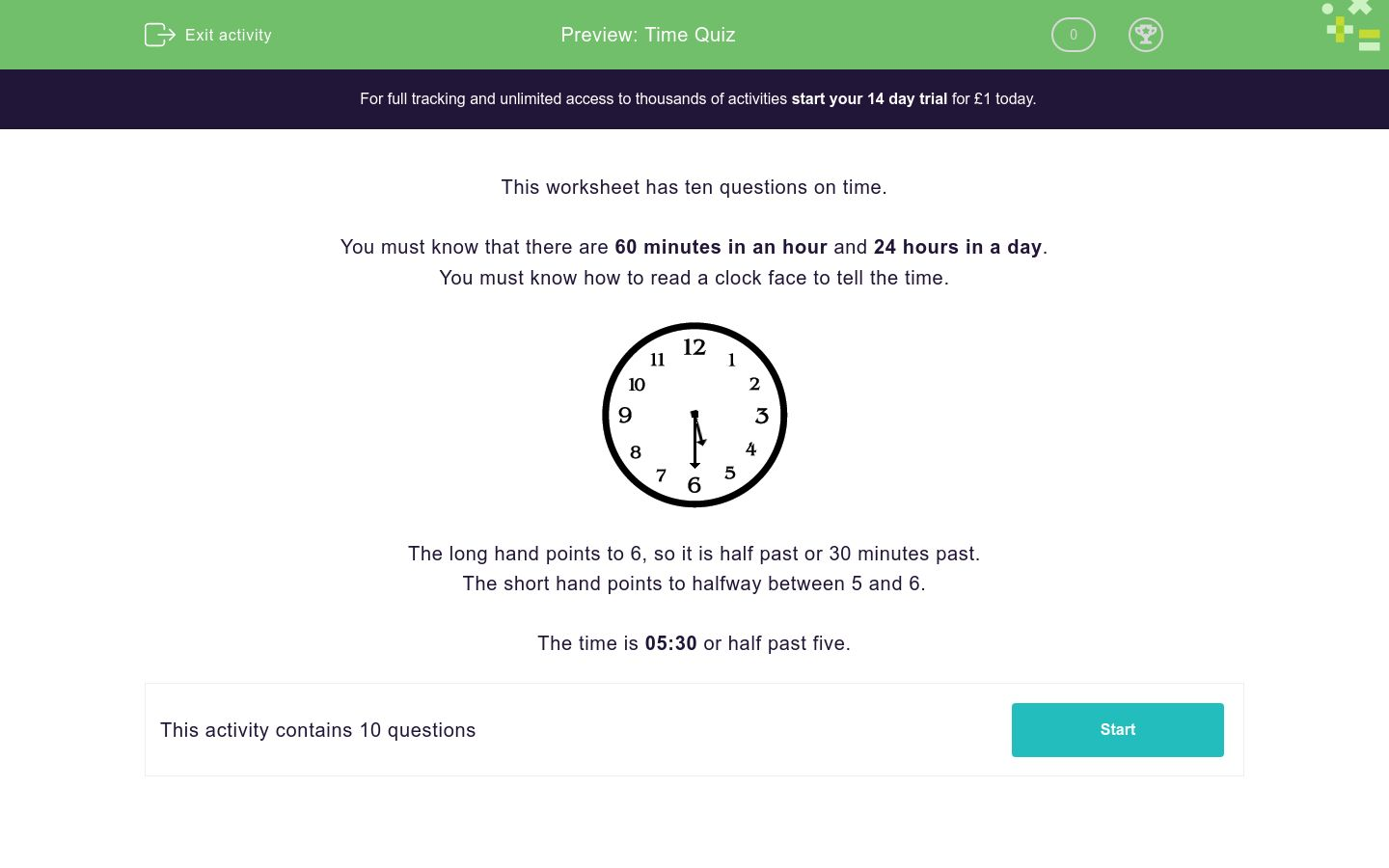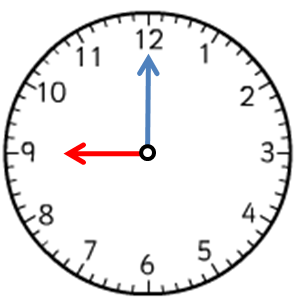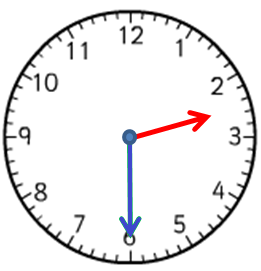# Time Quiz

In this worksheet, students must answer mixed questions related to time.Key stage:  KS 1

Curriculum topic:   Measurement

Curriculum subtopic:   Use Time Language

Difficulty level:### QUESTION 1 of 10

This worksheet has ten questions on time.

You must know that there are 60 minutes in an hour and 24 hours in a day.

You must know how to read a clock face to tell the time.The long hand points to 6, so it is half past or 30 minutes past.

The short hand points to halfway between 5 and 6.

The time is 05:30 or half past five.

What time is shown on the clock?12 noon

12:35

7 o'clock

07:12

What time is shown on the clock face??4 o'clock

O'clock past 9

Nine o'clock

4 past 30What time is shown on the clock face?

Half past 1.

1 o'clock

30 past

Half past 4

What time is shown on the clock?

Tick all the answers which are right.09:30

06:47

half past 9

ten to 6

What time is shown on the clock face?

Tick all of the correct answers.1 o'clock

2 o'clock

1:30

1:00

What time does the clock face show?

Tick all of the correct answersHalf past 1

Half past 2

1:30

2:30

How many minutes are there in an hour?

Which time shows midday?

Tick all the answers which could be right.

noon

midnight

12:00

12 o'clock

How many hours are there in a day?

What time is shown on the clock?06:35

06:30

half past 7

6 o'clock

• Question 1

What time is shown on the clock?7 o'clock
EDDIE SAYS
The long hand points to 12, so it is o'clock.
The short hand points to 7.
• Question 2

What time is shown on the clock face??Nine o'clock
EDDIE SAYS
The short hand points to the nine and the long hand points to the 12. We read this as nine o'clock or 9 o'clock.
• Question 3What time is shown on the clock face?

Half past 1.
EDDIE SAYS
The long hand points to the six. The short hand is half way between the one and the two. So, the time is half past one or 1:30.
• Question 4

What time is shown on the clock?

Tick all the answers which are right.09:30
half past 9
EDDIE SAYS
The long hand points to 6, so it is half past or 30 minutes past.
The short hand points to halfway between 9 and 10.
The time is 09:30 or half past nine.
• Question 5

What time is shown on the clock face?

Tick all of the correct answers.1 o'clock
1:00
EDDIE SAYS
The long hand points to the 12. The short hand points to the 1. The time is 1 o'clock.
• Question 6

What time does the clock face show?

Tick all of the correct answersHalf past 2
2:30
EDDIE SAYS
The long hand points to the 6. The short hand is halfway between the 2 and 3 so the time is half past 2 or 2:30.
• Question 7

How many minutes are there in an hour?

60
60 minutes
EDDIE SAYS
There are 60 minutes in an hour.
• Question 8

Which time shows midday?

Tick all the answers which could be right.

noon
12:00
12 o'clock
EDDIE SAYS
12:00 or 12 o'clock is midday or noon.
• Question 9

How many hours are there in a day?

24
24 hours
EDDIE SAYS
There are 24 hours in a whole day.
• Question 10

What time is shown on the clock?06:30
EDDIE SAYS
The long hand points to 6, so it is half past or 30 minutes past.
The short hand points to halfway between 6 and 7.
The time is 06:30 or half past six.
---- OR ----